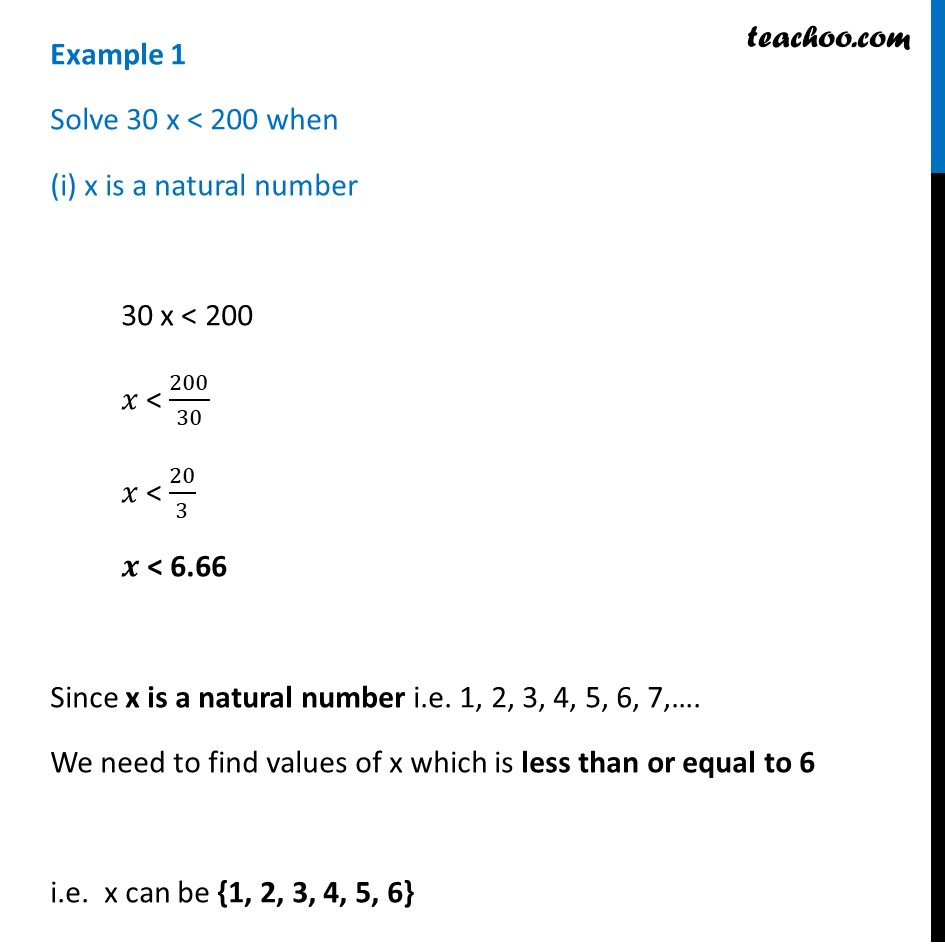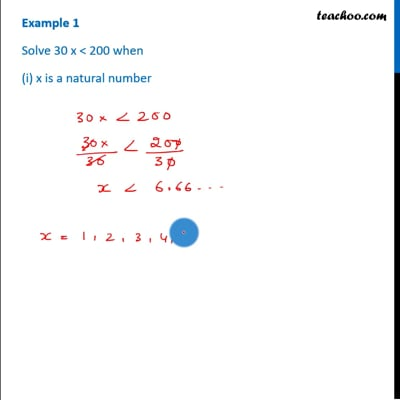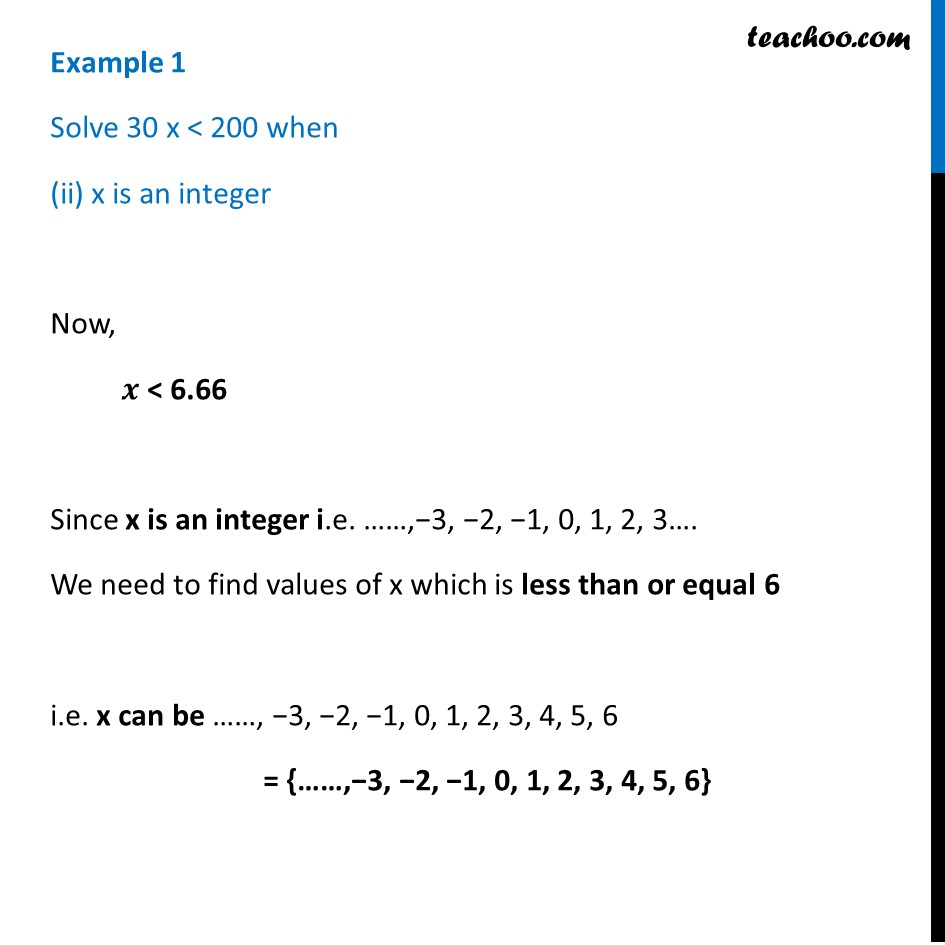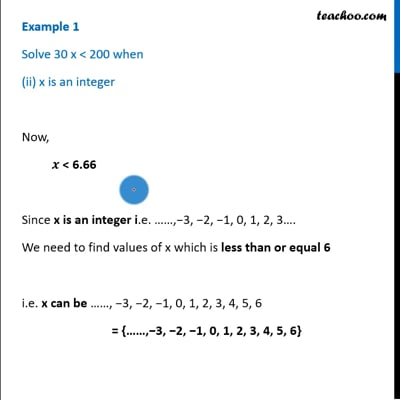Examples

Chapter 6 Class 11 Linear Inequalities (Term 2)
Serial order wiseThis video is only available for Teachoo black usersThis video is only available for Teachoo black users

### Transcript

Example 1 Solve 30 x < 200 when (i) x is a natural number 30 x < 200 𝑥 < 200/30 𝑥 < 20/3 𝒙 < 6.66 Since x is a natural number i.e. 1, 2, 3, 4, 5, 6, 7,…. We need to find values of x which is less than or equal to 6 i.e. x can be {1, 2, 3, 4, 5, 6} Example 1 Solve 30 x < 200 when (ii) x is an integer Now, 𝒙 < 6.66 Since x is an integer i.e. ……,−3, −2, −1, 0, 1, 2, 3…. We need to find values of x which is less than or equal 6 i.e. x can be ……, −3, −2, −1, 0, 1, 2, 3, 4, 5, 6 = {……,−3, −2, −1, 0, 1, 2, 3, 4, 5, 6}